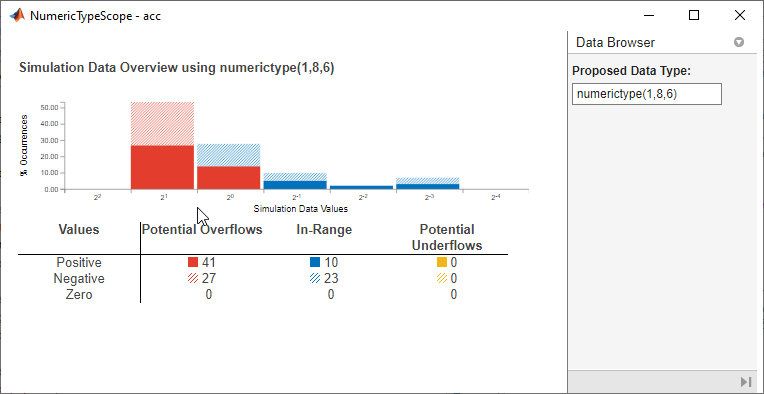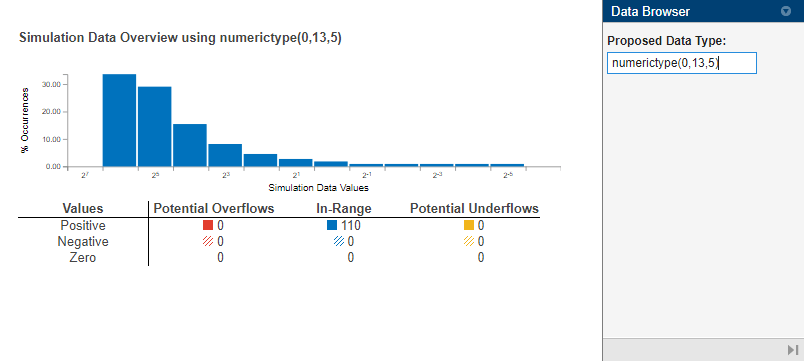# NumericTypeScope

Determine fixed-point data type

## Description

The `NumericTypeScope` object provides information about the dynamic range of your data. The scope provides a visual representation of the dynamic range of your data in the form of a `log2` histogram.

## Creation

### Syntax

``H = NumericTypeScope``

### Description

example

````H = NumericTypeScope` returns a `NumericTypeScope` object. After you create a `NumericTypeScope` object, use the `step` function to process your data and view the `NumericTypeScope`.```

The `NumericTypeScope` window visualizes the dynamic range of a `fi` object in a `log2` histogram. Bit weights appear along the x-axis of the histogram and the percentage of occurrences along the y-axis. Each bin of the histogram corresponds to a bit in the binary word. For example, 20 corresponds to the first integer bit in the binary word, and 2-1 corresponds to the first fractional bit in the binary word.

The `NumericTypeScope` identifies potential overflows and underflows based on the current data type. The scope indicates values that may cause overflow or underflow, or are in range of the data type by color-coding the histogram bars as follows:

• Blue — Histogram bin contains values that are in range of the current data type.

• Red — Histogram bin contains values that may cause overflow.

• Yellow — Histogram bin contains values that may cause underflow.

The table below the histogram breaks down each category of values by their signed value.

The Data Browser pane displays the current fixed-point data type as the Proposed Data Type. You can change the data type by entering a value directly in this box.## Object Functions

 `step` Process data and visualize dynamic range `show` Open `NumericTypeScope` object `reset` Clear stored information from `NumericTypeScope` object

## Examples

collapse all

Use the `NumericTypeScope` to view the dynamic range of a `fi` object.

Create a `fi` object and set the `DataTypeOverride` to `ScaledDoubles`.

```a = fi(magic(10),1,8,2); b = fi([a; 2.^(-5:4)],1,8,3); fp = fipref; initialDTOSetting = fp.DataTypeOverride; fp.DataTypeOverride = 'ScaledDoubles'; ```

Create a `NumericTypeScope` object. You can use the `reset` method to ensure that all stored information is cleared from the `NumericTypeScope` object `h`.

```h = NumericTypeScope; reset(h) ```

Use the `step` method to process your data and visualize the dynamic range of the `fi` object `b`.

```step(h,b); ```Closing the `NumericTypeScope` window does not delete the object from your workspace. Close the `NumericTypeScope` window and reopen it using the `show` function.

```show(h); ```

The `NumericTypeScope` displays a `log2` histogram which shows that the values appear both outside of the range and below the precision of the data type of the variable. Pause on one bar of the histogram to view the percentage of the total values that are represented by that bar.In this case, the data type of `b` is `numerictype(1,8,3)`. The `numerictype(1,8,3)` data type provides 5 integer bits, including the signed bit, and 3 fractional bits. Thus, this data type can represent only values between -2^4 and 2^4 - 2^-3 (from -16 to 15.8750). Given the range and precision of this data type, values greater than 2^4 fall outside the range and values less than 2^-3 fall below the precision of the data type.

The `NumericTypeScope` shows that values requiring bits 5, 6, and 7 are outside the range and values requiring fractional bits 4 and 5 are below precision. Given this information, you can prevent values that are outside range and below precision by changing the data type of the variable `b` to `numerictype(0,13,5)`.

Given this information, you can prevent values that are outside range and below precision by changing the data type of the variable `b` to `numerictype(0,13,5)`. In the `NumericTypeScope`, enter `numerictype(0,13,5)` in the Proposed Data Type box.```fp.DataTypeOverride = initialDTOSetting; ```

View the dynamic range and determine an appropriate numeric type for a `fi` object with a `DataTypeMode` of `Scaled double: binary point scaling`.

Create a `numerictype` object with a `DataTypeMode` of `Scaled double: binary point scaling`. Then, use that `numerictype` object to construct your `fi` objects.

```T = numerictype; T.DataTypeMode = 'Scaled double: binary point scaling'; T.WordLength = 8; T.FractionLength = 6; a = fi(sin(0:100)*3.5, T); b = fi(cos(0:100)*1.75,T); acc = fi(0,T); ```

Create a `NumericTypeScope` object `h`. Then, use the `step` function in a `for` loop to view the dynamic range of the accumulator object, `acc`.

```h = NumericTypeScope; for i = 1:length(a) acc(:) = a(i)*0.7+b(i); step(h,acc) end ```This dynamic range analysis shows that you can represent the entire range of data in the accumulator with 5 bits, two to the left of the binary point (integer bits) and three to the right of it (fractional bits). You can verify that this data type is able to represent all the values by changing the `WordLength` and `FractionLength` properties of the `numerictype` object `T`. Then, use `T` to redefine the accumulator.

```T.WordLength = 5; T.FractionLength = 2; acc = fi(0,T); ```

To view the dynamic range analysis based on this new data type, reset the `NumericTypeScope` object `h`, and rerun the loop.

```reset(h) for i = 1:length(a) acc(:) = a(i)*0.7 + b(i); step(h,acc) end ```Clear the information stored in the `NumericTypeScope` object `h`.

```reset(h); ```

## Version History

Introduced in R2010a

expand all